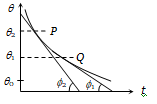• Subject:

...

• Topic:

...

A body cools in a surrounding which is at a constant temperature of ${\mathrm{\theta }}_{0}$. Assume that it obeys Newton's law of cooling. Its temperature $\mathrm{\theta }$ is plotted against time t. Tangents are drawn to the curve at the points P$\left(\mathrm{\theta }={\mathrm{\theta }}_{1}\right)$ and Q$\left(\mathrm{\theta }={\mathrm{\theta }}_{2}\right)$. These tangents meet the time axis at angles of ${\mathrm{\varphi }}_{2}$ and ${\mathrm{\varphi }}_{1}$, as shown(a) $\mathrm{tan}\frac{{\mathrm{\varphi }}_{2}}{{\mathrm{\varphi }}_{1}}=\frac{{\mathrm{\theta }}_{1}-{\mathrm{\theta }}_{0}}{{\mathrm{\theta }}_{2}-{\mathrm{\theta }}_{0}}$                          (b) $\mathrm{tan}\frac{{\mathrm{\varphi }}_{2}}{{\mathrm{\varphi }}_{1}}=\frac{{\mathrm{\theta }}_{2}-{\mathrm{\theta }}_{0}}{{\mathrm{\theta }}_{1}-{\mathrm{\theta }}_{0}}$
(c) $\mathrm{tan}\frac{{\mathrm{\varphi }}_{1}}{{\mathrm{\varphi }}_{2}}=\frac{{\mathrm{\theta }}_{1}}{{\mathrm{\theta }}_{2}}$                                 (d) $\mathrm{tan}\frac{{\mathrm{\varphi }}_{1}}{{\mathrm{\varphi }}_{2}}=\frac{{\mathrm{\theta }}_{2}}{{\mathrm{\theta }}_{1}}$